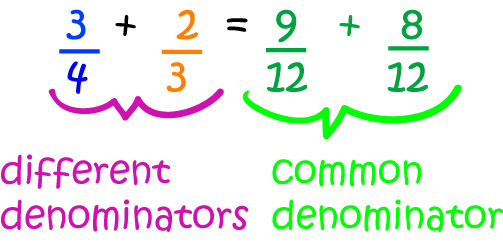# Definition of Least Common DenominatorThe least common denominator of a group of $2$ or more fractions is the smallest number that can be used as the denominator of all of the fractions in the group.

Just a few reminders:

• The "denominator" is the number on the bottom of a fraction.
• A "common denominator" occurs when the bottom number of all of the fractions in the group is the same.
• The "least common denominator" is the smallest out of all of the positive numbers that can be used as common denominators for all of the fractions in the group.

For example, let's find the least common denominator of the fractions $\dfrac{1}{4}$ and $\dfrac{2}{3}$.

$\dfrac{1}{4}$ can be written as $\dfrac{1}{4}$, $\dfrac{2}{8}$, $\dfrac{3}{12}$, $\dfrac{4}{16}$, and so on.

$\dfrac{2}{3}$ can be written as $\dfrac{4}{6}$, $\dfrac{8}{12}$, $\dfrac{12}{18}$, and so on.

The first fractions from the two lists that have the same denominator are $\dfrac{3}{12}$ and $\dfrac{8}{12}$. So, the least common denominator of $\dfrac{1}{4}$ and $\dfrac{3}{12}$ is $12$.

Finding the least common denominator makes it much easier to add and subtract fractions.

For example,

$\dfrac{1}{4} + \dfrac{2}{3} = \dfrac{3}{12} + \dfrac{8}{12} = \dfrac{11}{12}.$

### Description

The aim of this dictionary is to provide definitions to common mathematical terms. Students learn a new math skill every week at school, sometimes just before they start a new skill, if they want to look at what a specific term means, this is where this dictionary will become handy and a go-to guide for a student

### Audience

Year 1 to Year 12 students

### Learning Objectives

Learn common math terms starting with letter L

Author: Subject Coach
You must be logged in as Student to ask a Question.